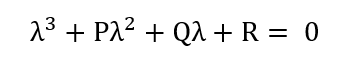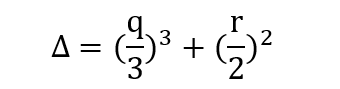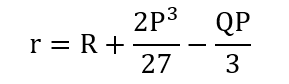# Calculate Delta Criterion

Problem:

How do I calculate Delta Criterion?

Solution:

The easiest way to do this is to use a Tecplot Macro to automate the calculation. Calculations are derived from https://www.ctr.maths.lu.se/media/thesis/2013/FMA820.pdf

First, make sure Tecplot has established the velocity variables via the Field Variables dialog (Analyze -> Field Variables). Then, play the macro via the Scripting menu.

The macro commands are as follows:

• Calculate the Velocity Gradient tensor. (Can also be calculated via Analyze->Calculate Variables…->Velocity Gradient (tensor))
• The characteristic equation of ∇u is:• Use the Specify Equations dialog to computer P, Q and R:

\$!ALTERDATA EQUATION = '{P} = -({dUdX}+{dVdY}+{dWdZ})' #0 for incompressible flows
\$!ALTERDATA EQUATION = '{Q} = (-{dUdY}*{dVdX}-{dUdZ}*{dWdX}-{dVdZ}*{dWdY}+{dUdX}*{dVdY}+{dWdZ}*{dUdX}+{dWdZ}*{dVdY})'
\$!ALTERDATA EQUATION = '{R} = ({dUdX}*({dVdZ}*{dWdY}-{dVdY}*{dWdZ})+{dUdY}*({dVdX}*{dWdZ}-{dWdX}*{dVdZ})+{dUdZ}*({dWdX}*{dVdY}-{dVdx}*{dWdY}))'

• The delta criterion is the discriminant of the characteristic equation of ∇uWhere:to account for compressible effects (P not equal to 0).